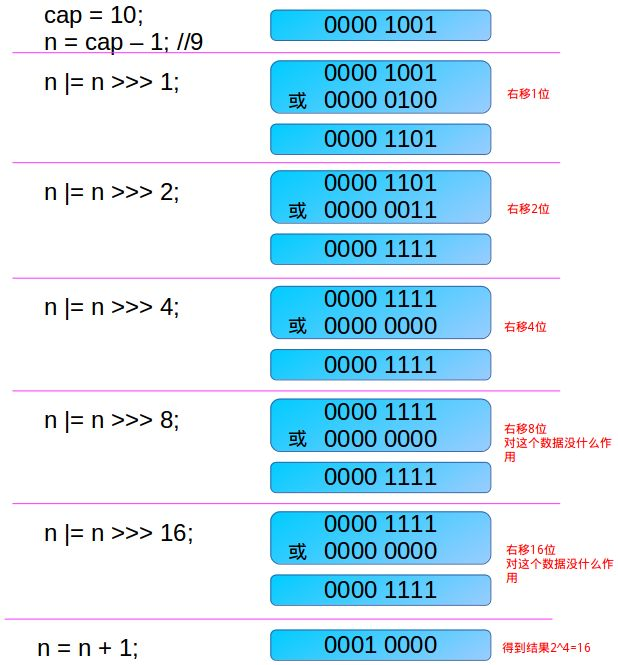/**
* Returns a power of two size for the given target capacity.
* 返回大于或等于 cap 的最小2次幂
*/
static final int tableSizeFor(int cap) {
int n = cap - 1;
n |= n >>> 1;
n |= n >>> 2;
n |= n >>> 4;
n |= n >>> 8;
n |= n >>> 16;
return (n < 0) ? 1 : (n >= MAXIMUM_CAPACITY) ? MAXIMUM_CAPACITY : n + 1;
}

1、数据在内存中如何存储？

00000000 00000000 00000000 00001010 ->对应 1x2^3+0x2^2+1x2^1+0x2^0 =10

11111111 11111111 11111111 11111111 ->对应 1x2^31+1x2^30…1x2^1+1x2^0

11111111 11111111 11111111 11111111 所代表的数字为：1x2^30+1x2^29…1x2^1+1x2^0 的相反数为：-(2^31-1)

00000000 00000000 00000000 00000000 为+0

10000000 00000000 00000000 00000000 为-0

2、位运算如何进行？

• 对于符号位移

10000000 00000000 00000000 0010100 为-20

• 对于无符号位移

010000000 00000000 00000000 0000101 变成了一个很大的正数了！！

00000000 00000000 00000000 00001010 = 20

3、关于返回大于或等于 cap 的最小2次幂的算法